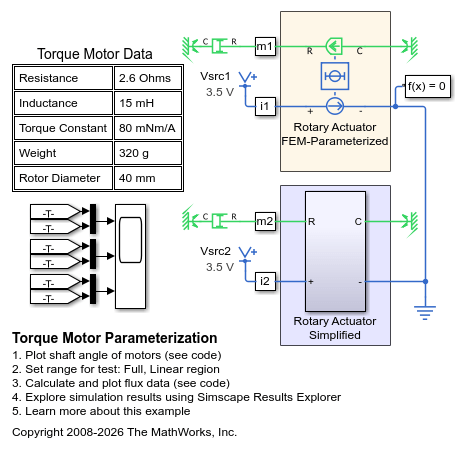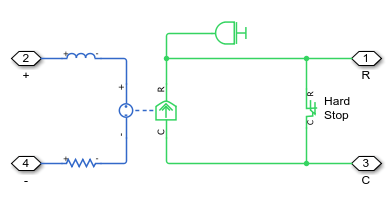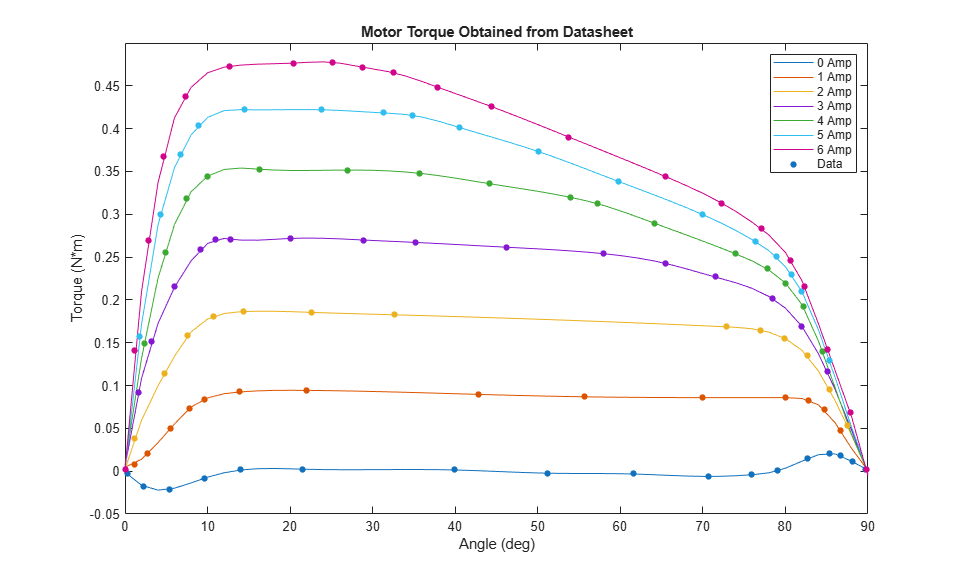# Torque Motor Parameterization

This example shows how manufacturer data for torque as a function of current and angle can be used to model a torque motor. The datasheet shows linear characteristics for rotor angles between 20 and 70 degrees and for currents where saturation does not occur. Data in this range is used to parameterize the simplified model of the torque motor. Using MATLAB® to process the data points extracted from the datasheet, we can convert manufacturer data into motor parameters that are often obtained from finite element software.

The motor models show similar results when tested under conditions where the datasheet shows linear behavior. When tested over the full range, the behaviors deviate as specified in the datasheet.

### Model### Rotary Actuator Simplified Subsystem### Obtaining Motor Data from Data Sheet

The plot below shows resampled data obtained from a motor data sheet. It shows torque produced at different rotor angles at different current levels. For some conditions (such as 2 amps, 20 deg to 70 deg), the torque is constant, but at other levels it is highly nonlinear.To parameterize our motor model, we need to obtain flux partial derivative with respect to angle. This script estimates dPhi/dx from torque. First, we mirror the datasheet to obtain data for negative currents and plot it as a surface.Next, we use MATLAB to fit polynomial curves to the surface along lines of constant angle.Finally, we use MATLAB to obtain the derivative of the polynomial along those curves. Extracting a lookup table from this surface yields the parameters we need for our motor model.### Simulation Results from Simscape Logging

The plot below shows the behavior of the FEM-Parameterized Rotary Actuator and simplified model built from Simscape™ Foundation Library elements. This test was performed over the range of travel where the finite-element data is linear, so the results are similar.Performing the test over wider range over the range of travel where the finite-element data is nonlinear shows the effect of our parameterization as two motors behave very differently.### Results from Real-Time Simulation

This example has been tested on a Speedgoat Performance real-time target machine with an Intel® 3.5 GHz i7 multi-core CPU. This model can run in real time with a step size of 50 microseconds.